Email us to get an instant 20% discount on highly effective K-12 Math & English kwizNET Programs!

#### Online Quiz (WorksheetABCD)

Questions Per Quiz = 2 4 6 8 10

### Grade 1 - Mathematics3.20 Counting 100s 10s and 1s with Pictures

 Method: Count the number of hundreds. Count the number of tens. Count the number of units. Add all of them. Example:Count the number of pictures and accordingly write the number. Number of hundreds is 4 = 400 Number of Tens is 1 = 10 Number of Ones is 2 = 2 By counting we get 400 + 10 + 2 = 412 Answer:412 Directions: Answer the following questions. Also write at least five examples of your own.
 Q 1: Using the picture count by 100s 10s and 1s.Answer: Q 2: Using the picture count by 100s 10s and 1s.Answer: Q 3: Using the picture count by 100s 10s and 1s.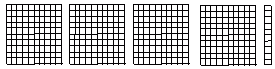Answer: Q 4: Using the picture count by 100s 10s and 1s.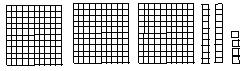Answer: Q 5: Using the picture count by 100s 10s and 1s.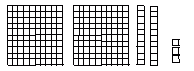Answer: Q 6: Using the picture count by 100s 10s and 1s.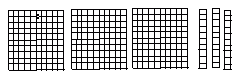Answer: Q 7: Using the picture count by 100s 10s and 1s.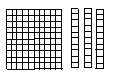Answer: Q 8: Using the picture count by 100s 10s and 1s.Answer: Question 9: This question is available to subscribers only! Question 10: This question is available to subscribers only!